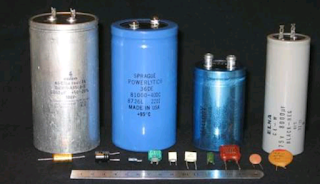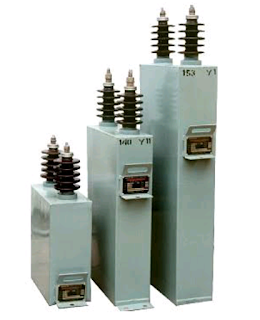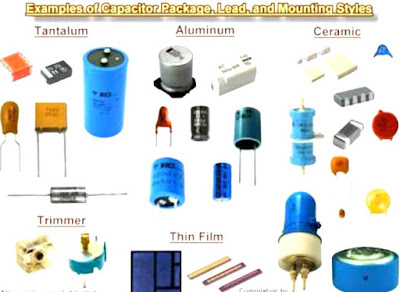# Basic Knowledge Of Electrical Capacitor | About The Electrical Components Which Mostly Use In Electrical Application!!

Basic Knowledge Of  Electrical Capacitor |  About The Electrical Components Which Mostly Use In Electrical Application!!

Get The Basic Knowledge Of Electrical Capacitor And Description For The Electrical Component - Electrical capacitor Which Are The Most Useful Device In Electrical Basic Circuits.

Aa Capacitor is a passive two-terminal Electrical Component that stores Electrical Energy in an Electric Field.

The effect of aa capacitor is known as Capacitance

A While capacitance exists between any two Electrical Conductors of a circuit in sufficiently close proximity,

A Capacitor is specifically designed to provide and enhance this effect for a variety of practical applications by consideration of size, shape, and positioning of closely spaced conductors, and the intervening Dielectric Material.

Recommended Articles :

We can understand the working of a capacitor by using the water tank analogy.

• Imagine a water tank with fixed base area and height. The volume of the tank is the capacity of the tank to store water.
• The height of the water in the water tank decides the potential energy of the water. For the same volume of water stored, a tank with lower base area and more height contains water with more potential energy than a tank with large base area and less height.
• The rate of filling of the tank depends on the rate at which the water is poured into the tank.
• If there is a leakage in the tank, some water keeps getting out of the tank, which is a wastage or loss.
Now, let's look at the capacitor:
• The capacity to store charges is like the capacity of the tank to store water.
• The voltage rating of the capacitor is analogous to the height of the water tank. A higher voltage rating ensures that the potential energy of the capacitor is more when fully charged [ Realation Between Electrical Charge And Electrical Current  ].
• The rate of charging in a capacitor depends on the rate of flow of charges, which is current. It is analogous to the rate of flow of water into the tank.
• All capacitors are lossy, which means that a charged capacitor subsequently loses its charge by slowly dissipating energy through it's loss component, which is a resistive component of a capacitor.

Capacitors are Energy storing elements, which store energy in the form of an electric field.

When subjected to a voltage, a capacitor draws Current until the potential reaches the voltage rating of the capacitor.

If a higher potential is applied, it may result in damage of the capacitor.

The energy stored is directly proportional to the square of the voltage across the capacitor.

Aa capacitor was  Historically [ History Of Electrical Engineering  ]  first known as an Electric Condenser.

The earliest capacitors were glass jars, metalised in and out.

they were made by Pieter van Musschenbroek of Leyden, Holland , who was exploiting a phenomenon observed the previous year by Ewald G. von Kleist, using simple medicine bottles.

Musschenbroek had been generating sparks and shocks with a static electricity machine, and used the jars as a medium term way of storing and transferring the effect.

This was 1746 and the jars became known as Leyden jars, presumably because no-one could spell Musschenbroek.

initially capacitance was measured in Jars, and the British navy was still doing that until after the second world war.

One RN Jar was about 1111pF. The size of the Jar (as a unit) had varied a bit, up to about 1910.

Glass jars are very good capacitors with a high breakdown voltage and low self-discharge

Type :- Passive

Invented :- Ewald Georg von Kleist

The physical form and construction of practical a capacitors vary widely and many capacitor types are in common use.

Aa Most capacitors contain at least two Electrical Conductors often in the form of metallic plates or surfaces separated by a dielectric medium.

Aa conductor may be a foil, thin film, sintered bead of metal, or an electrolyte. The nonconducting dielectric acts to increase the capacitor's Charge capacity.

i

Aa Materials commonly used as dielectrics include glass, ceramic, plastic film, paper, mica, and oxide layers.

Capacitors are widely used as parts of Electrical Circuits in many common Electrical Devices.

Unlike aa Resistor, an ideal capacitor does not dissipate energy.Exampl Of Capacitors

When two conductors experience aa potential difference,

for example, when a capacitor is attached across a battery,

an Electric Field develops across the dielectric, causing a net positive charge to collect on one plate and net negative charge to collect on the other plate.
• A No current actually flows through the dielectric, however, there is a flow of charge through the source circuit.

If the condition is maintained sufficiently long, the current through the source circuit ceases.

However, if aa time-varying voltage is applied across the leads of the capacitor,

the source experiences an ongoing current due to the charging and discharging cycles of the capacitor.Electrical Capacitor

Capacitance is defined as a  the ratio of the electric charge on each conductor to the Potential Difference between them.

The unit of aa capacitance in the International System of Units (SI) is the farad (F), defined as one coulomb per volt (1 C/V).

A Capacitance values of typical capacitors for use in general electronics range from about 1 picofarad (pF) (10−12 F) to about 1 millifarad (mF) (10−3 F).

The capacitance of aa capacitor is proportional to the surface area of the plates (conductors) and inversely related to the gap between them

In practice, the dielectric between the plates passes aa small amount of leakage current.

It has an a electric field strength limit, known as the breakdown voltage.

The conductors and a leads introduce an undesired inductance and resistance.Example Of Electrical Capacitors

Aa Capacitors are widely used in electronic circuits for blocking direct current while allowing alternating current to pass.

Aa In analog filter networks, they smooth the output of power supplies.

Aa In resonant circuits they tune radios to particular frequencies.

Aa In electric power transmission systems, they stabilize voltage and power flow.

The property of energy storage in capacitors was exploited as dynamic memory in early digital computers aa.

You May Like To Read This Related Articles :

From This Article You Can Solve Below Queries :

### What is capacitor and how it works What is the principle of a capacitor What is the basic use of capacitor? Why would you use a capacitor?

#### 1 comment:

1.Extremely useful information which you have shared here about Non-Metallic Boxes. This is a great way to enhance knowledge for us, and also helpful for us. Thankful to you for sharing an article like this.